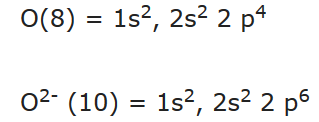Filling of Orbitals in Atom

The filling of electrons into the orbitals of different atoms takes place according to the aufbau principle which is based on the Pauli’s exclusion principle, the Hund’s rule of maximum multiplicity and the relative energies of the orbitals.

# Aufbau Principle

• The word ‘aufbau’ in German means ‘building up’. The building up of orbitals means the filling up of orbitals with electrons. The principle states: In the ground state of the atoms, the orbitals are filled in order of their increasing energies.
• In other words, electrons first occupy the lowest energy orbital available to them and enter into higher energy orbitals only when the lower energy orbitals are filled.
• Unlike H-atom where energy of orbitals depends only on n, energy of orbitals of multi-electron orbitals depend on both n and l. Their order of energy can be determined using (n+l) rule.
• According to this rule, lower the value of (n+l) for an orbital, lower is its energy. If two different types of orbitals have the same value of (n+l), the orbital with lower value of n has lower energy.# Hund’s rule of maximum multiplicity

• This rule deals with the filling of electrons into the orbitals belonging to the same subshell (that is, orbitals of equal energy, called degenerate orbitals).
• It states: the pairing of electrons in the orbitals belonging to the same subshell (p, d or f) does not take place until each orbital belonging to that subshell has got one electron each i.e., it is singly occupied.

# Pauli Exclusion Principle:

• According to the principle, no two electrons in an atom can have the same set of four quantum numbers.
• Pauli exclusion principle can also be stated as: Only two electrons may exist in the same orbital and these electrons must have opposite spins.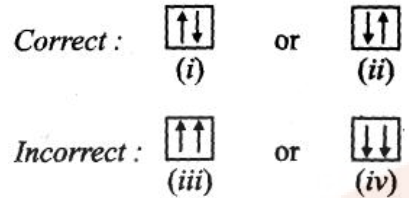• The maximum number of electrons in the shell with principal quantum number n is equal to 2n2.

# Electronic Configuration of Atoms

• The distribution of electrons into orbitals of an atom is called electronic configuration.
• The electronic configuration of different atoms can be represented in two ways.

For example:

1. sa pb dc … notation
2. Orbital diagram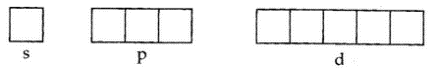For example: The electronic configuration of hydrogen 1s1.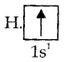• Causes of stability of Completely Filled and Half-Filled Subshells

The completely filled and half-filled subshells are stable due to the following reasons: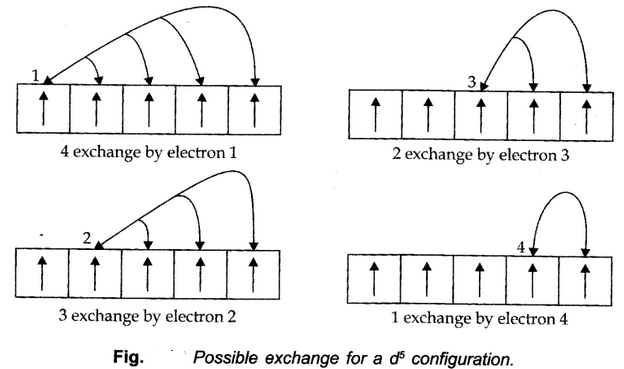1. Symmetrical distribution of electrons: The completely filled or half-filled subshells have a symmetrical distribution of electrons in them and are therefore more stable.
2. The stabilizing effect arises whenever two or more electrons with same spin are present in the degenerate orbitals of a subshell. These electrons tend to exchange their positions and the energy released due to their exchange is called exchange energy. The number of exchanges that can takes place is maximum when the subshell is either half-filled or completely filled.

# Methods of writing Electronic Configuration

## Orbital method:

In this, the electrons present in respective orbitals are denoted.

E.g. Cl (17) = 1s2, 2s2, 2p6, 3s2, 2p5.

## Shell method:

In this, the number of electrons in each shell is continuously written.

e.g. Cl (17) =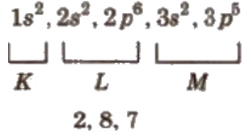## Box method:

In this method, each orbital is denoted by a box and electrons are represented by half-headed (↑) or full-headed (↑) arrows. An orbital can occupy a maximum of two electrons.

e.g.# Electronic Configuration of Ions

To write the electronic configuration of ions. First, write the electronic configuration of neutral atom and then add (for a negative charge) or remove (for a positive charge) electrons in outer shell according to the nature and magnitude of charge present on the ion.

e.g.